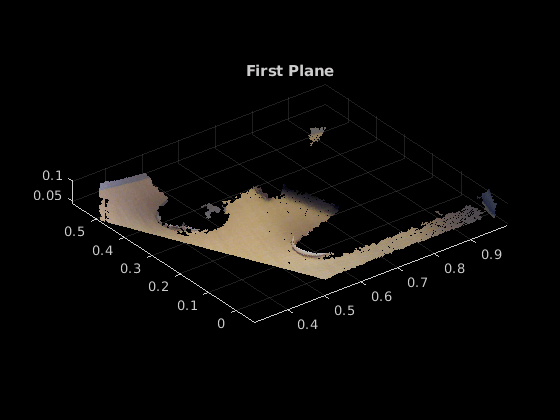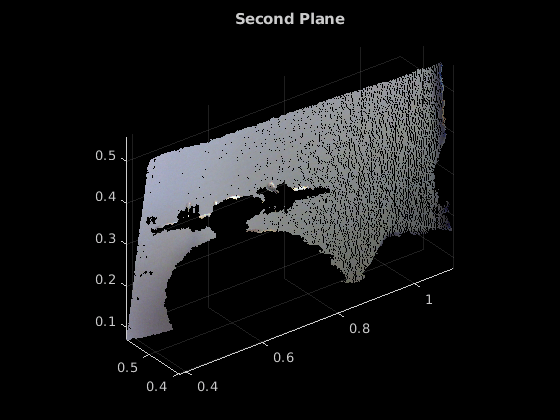# planeModel

Object for storing a parametric plane model

## Description

Construct and store a parametric plane model based on parameters that describe a plane.

## Creation

### Syntax

``model = planeModel(Parameters)``

### Description

example

````model = planeModel(Parameters)` constructs a parametric plane model from the 1-by-4 `Parameters` input vector that describes a plane. ```

### Input Arguments

expand all

Plane parameters, specified as a 1-by-4 vector. This input specifies the `Parameters` property. The four parameters [a,b,c,d] describe the equation for a plane:

`$ax+by+cz+d=0$`

## Properties

expand all

Plane model parameters, stored as a 1-by-4 vector. These parameters are specified by the `Parameters` input argument.

Normal vector of the plane, stored as a 1-by-3 vector. The [a,b,c] vector specifies the unnormalized normal vector of the plane.

## Object Functions

 `plot` Plot parametric model `normalRotation` Compute transform for rotation of a normal to a plane

## Examples

collapse all

`load("object3d.mat")`

Display and label the point cloud.

```figure pcshow(ptCloud) xlabel("X(m)") ylabel("Y(m)") zlabel("Z(m)") title("Original Point Cloud")```Set the maximum point-to-plane distance (2cm) for plane fitting.

`maxDistance = 0.02;`

Set the normal vector of the plane.

`referenceVector = [0,0,1];`

Set the maximum angular distance to 5 degrees.

`maxAngularDistance = 5;`

Detect the first plane, the table, and extract it from the point cloud.

```[model1,inlierIndices,outlierIndices] = pcfitplane(ptCloud,... maxDistance,referenceVector,maxAngularDistance); plane1 = select(ptCloud,inlierIndices); remainPtCloud = select(ptCloud,outlierIndices);```

Set the region of interest to constrain the search for the second plane, left wall.

```roi = [-inf,inf;0.4,inf;-inf,inf]; sampleIndices = findPointsInROI(remainPtCloud,roi);```

Detect the left wall and extract it from the remaining point cloud.

```[model2,inlierIndices,outlierIndices] = pcfitplane(remainPtCloud,... maxDistance,SampleIndices=sampleIndices); plane2 = select(remainPtCloud,inlierIndices); remainPtCloud = select(remainPtCloud,outlierIndices);```

Plot the two planes and the remaining points.

```figure pcshow(plane1) title("First Plane")``````figure pcshow(plane2) title("Second Plane")``````figure pcshow(remainPtCloud) title("Remaining Point Cloud")```## Version History

Introduced in R2015b